## 3.14Linear upwind scheme

Linear upwind is another signiﬁcant scheme for advection. It is used particularly for advection of momentum, i.e.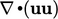or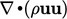but can be eﬀective for advection of other variables.The scheme reduces the diﬀusive nature of upwind by including additional upwind cell values indirectly from the gradient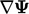in the upwind cell.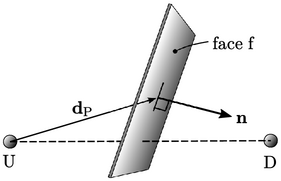Linear upwind describes the face value as an extrapolation of the upwind cell value to the face using the upwind cell gradient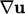and a vector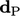from the cell centre to face centre. It ﬁrst provides a contribution to the coeﬃcients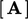of a matrix equation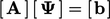by representing face values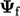by the upwind value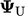. The extrapolation is then introduced through an additional explicit contribution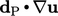to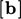.

### Skewness

Interpolation of values between cell centres is along a line joining the cell centres. Any interpolated value at a face relates to the point on the face intersected by that line.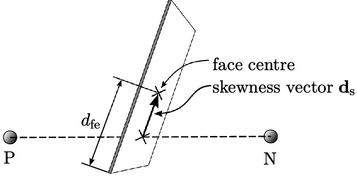Skewness is the distance between the intersection point and the face centre. It can be represented by a vector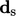or as the ratio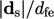, where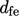is the distance from the face centre to edge in the direction of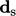.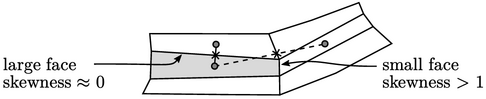An interpolated value represents an average across a face, but as skewness increases, this representation becomes less accurate. High skewness (e.g.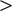1) does not immediately equate to poor accuracy, however, since interpolated values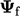are multiplied by face areas within a surface integral, e.g.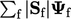— and high skewness occurs at small faces.

Advection schemes do not generally include a correction for high skewness to improve accuracy. However, the linear upwind scheme naturally includes skewness correction since the explicit contribution is in the direction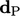towards the face centre.

Notes on CFD: General Principles - 3.14 Linear upwind scheme